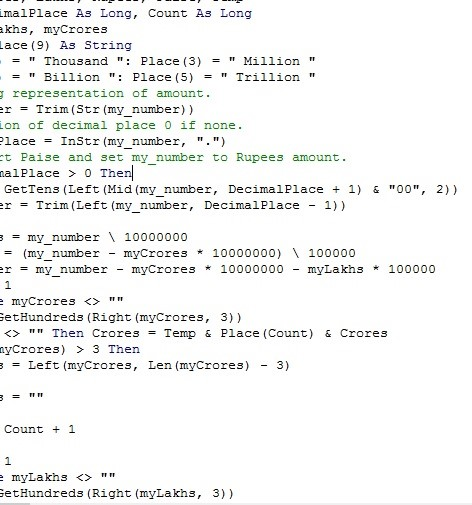# Excel vba, Convert Amount value Number to word, convert inr value in word

Educations 17 Thu 07 Jul 2022### How convert INR value from number to word in excel vba

##### open excel file , activate developer tool, under developer tool below in file menu showing visual basic,click visual basic editor and insert modulein modul create function with your choice or  below code copy and paste it module file and save as micro enable file.

code saction below

Function bestsaller_num_word(ByVal my_number, Optional incRupees As Boolean = True)
Dim Crores, Lakhs, Rupees, Paise, Temp
Dim DecimalPlace As Long, Count As Long
Dim myLakhs, myCrores
ReDim Place(9) As String
Place(2) = " Thousand ": Place(3) = " Million "
Place(4) = " Billion ": Place(5) = " Trillion "
' String representation of amount.
my_number = Trim(Str(my_number))
' Position of decimal place 0 if none.
DecimalPlace = InStr(my_number, ".")
' Convert Paise and set my_number to Rupees amount.
If DecimalPlace > 0 Then
Paise = GetTens(Left(Mid(my_number, DecimalPlace + 1) & "00", 2))
my_number = Trim(Left(my_number, DecimalPlace - 1))
End If
myCrores = my_number \ 10000000
myLakhs = (my_number - myCrores * 10000000) \ 100000
my_number = my_number - myCrores * 10000000 - myLakhs * 100000
Count = 1
Do While myCrores <> ""
Temp = GetHundreds(Right(myCrores, 3))
If Temp <> "" Then Crores = Temp & Place(Count) & Crores
If Len(myCrores) > 3 Then
myCrores = Left(myCrores, Len(myCrores) - 3)
Else
myCrores = ""
End If
Count = Count + 1
Loop
Count = 1
Do While myLakhs <> ""
Temp = GetHundreds(Right(myLakhs, 3))
If Temp <> "" Then Lakhs = Temp & Place(Count) & Lakhs
If Len(myLakhs) > 3 Then
myLakhs = Left(myLakhs, Len(myLakhs) - 3)
Else
myLakhs = ""
End If
Count = Count + 1
Loop
Count = 1
Do While my_number <> ""
Temp = GetHundreds(Right(my_number, 3))
If Temp <> "" Then Rupees = Temp & Place(Count) & Rupees
If Len(my_number) > 3 Then
my_number = Left(my_number, Len(my_number) - 3)
Else
my_number = ""
End If
Count = Count + 1
Loop
Select Case Crores
Case "": Crores = ""
Case "One": Crores = " One Crore "
Case Else: Crores = Crores & " Crores "
End Select
Select Case Lakhs
Case "": Lakhs = ""
Case "One": Lakhs = " One Lakh "
Case Else: Lakhs = Lakhs & " Lakhs "
End Select
Select Case Rupees
Case "": Rupees = "Zero "
Case "One": Rupees = "One "
Case Else:

Rupees = Rupees
End Select
Select Case Paise
Case "": Paise = " and Paise Zero Only "
Case "One": Paise = " and Paise One Only "
Case Else: Paise = " and Paise " & Paise & " Only "
End Select
NUM_TO_IND_RUPEE_WORD = IIf(incRupees, "Rupees ", "") & Crores & _
Lakhs & Rupees & Paise
End Function

' Converts a number  100 to 999 into text
Function GetHundreds(ByVal my_number)
Dim Result As String
If Val(my_number) = 0 Then Exit Function
my_number = Right("000" & my_number, 3)
' Convert in hundreds .
If Mid(my_number, 1, 1) <> "0" Then
Result = GetDigit(Mid(my_number, 1, 1)) & " Hundred "
End If
' Convert the tens and ones place.
If Mid(my_number, 2, 1) <> "0" Then
Result = Result & GetTens(Mid(my_number, 2))
Else
Result = Result & GetDigit(Mid(my_number, 3))
End If
GetHundreds = Result
End Function

' Converts   10 to 99 in text.
Function GetTens(TensText)
Dim Result As String
Result = "" ' Null out the temporary function value.
If Val(Left(TensText, 1)) = 1 Then ' If value between 10-19...
Select Case Val(TensText)
Case 10: Result = "Ten"
Case 11: Result = "Eleven"
Case 12: Result = "Twelve"
Case 13: Result = "Thirteen"
Case 14: Result = "Fourteen"
Case 15: Result = "Fifteen"
Case 16: Result = "Sixteen"
Case 17: Result = "Seventeen"
Case 18: Result = "Eighteen"
Case 19: Result = "Nineteen"
Case Else
End Select
Else ' If value between 20-99...
Select Case Val(Left(TensText, 1))
Case 2: Result = "Twenty "
Case 3: Result = "Thirty "
Case 4: Result = "Forty "
Case 5: Result = "Fifty "
Case 6: Result = "Sixty "
Case 7: Result = "Seventy "
Case 8: Result = "Eighty "
Case 9: Result = "Ninety "
Case Else
End Select
Result = Result & GetDigit _
(Right(TensText, 1)) ' Retrieve ones place.
End If
GetTens = Result
End Function
' Converts a number  1 - 9 into text.
Function GetDigit(Digit)
Select Case Val(Digit)
Case 1: GetDigit = "One"
Case 2: GetDigit = "Two"
Case 3: GetDigit = "Three"
Case 4: GetDigit = "Four"
Case 5: GetDigit = "Five"
Case 6: GetDigit = "Six"
Case 7: GetDigit = "Seven"
Case 8: GetDigit = "Eight"
Case 9: GetDigit = "Nine"
Case Else: GetDigit = ""
End Select
End Function

endcode saction

you can convert any number value in word

like  Range("b1")=bestsaller_num_word("A1")

if range("A1") valie is 123  than  range('b1") value will be " one hundred twenty three rupees"

--Posted By : superon